# Combine Table & Plot in Same Graphic Layout in R (Example)

In this tutorial, I’ll illustrate how to arrange plots and tables in the same grid layout in the R programming language.

The tutorial is structured as follows:

Let’s get started…

## Example Data, Software Packages & Default Plot

The first step is to define some data that we can use in the examples later on:

```data <- data.frame(x = 1:9,                         # Create example data frame
y = c(1, 5, 2, 5, 5, 2, 1, 2, 1),
group = LETTERS[1:3])
data                                                # Print example data frame```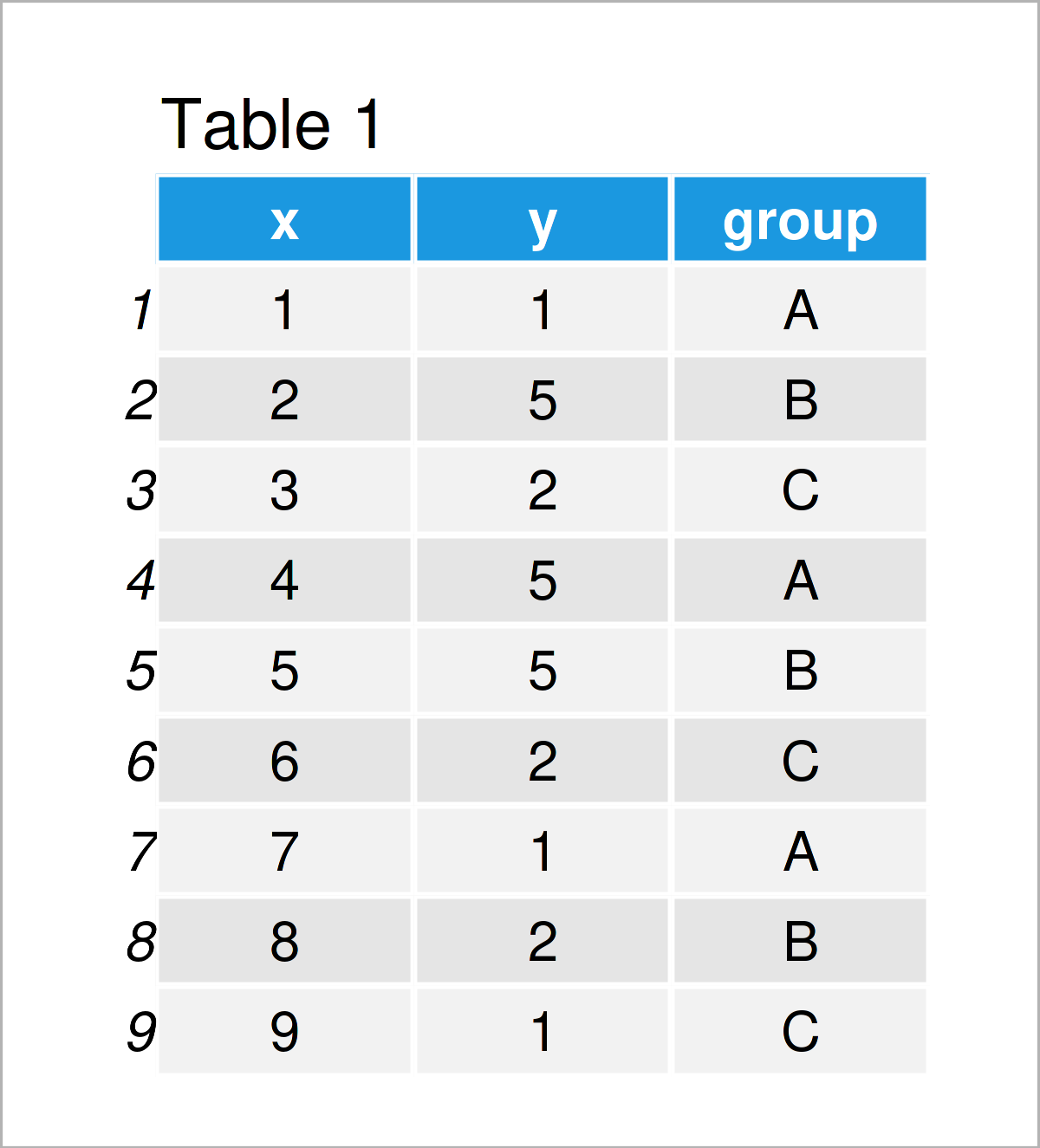Have a look at the previous table. It shows that our example data consists of nine rows and three variables.

Next, I’ll explain how to draw a grid of plots and tables based on these data.

## Example: Draw Plot & Table Using ggplot2, ggpmisc & patchwork Packages

In this example, I’ll demonstrate how to combine multiple plots and tables in the same graphical layout side-by.side.

For this example, we first need to install and load the ggplot2 package:

```install.packages("ggplot2")                         # Install & load ggplot2
library("ggplot2")```

Now, we can draw our data as shown below:

```ggp <- ggplot(data,                                 # Create ggplot2 plot
aes(x = x,
y = y,
color = group)) +
geom_point(size = 3)
ggp                                                 # Draw ggplot2 plot```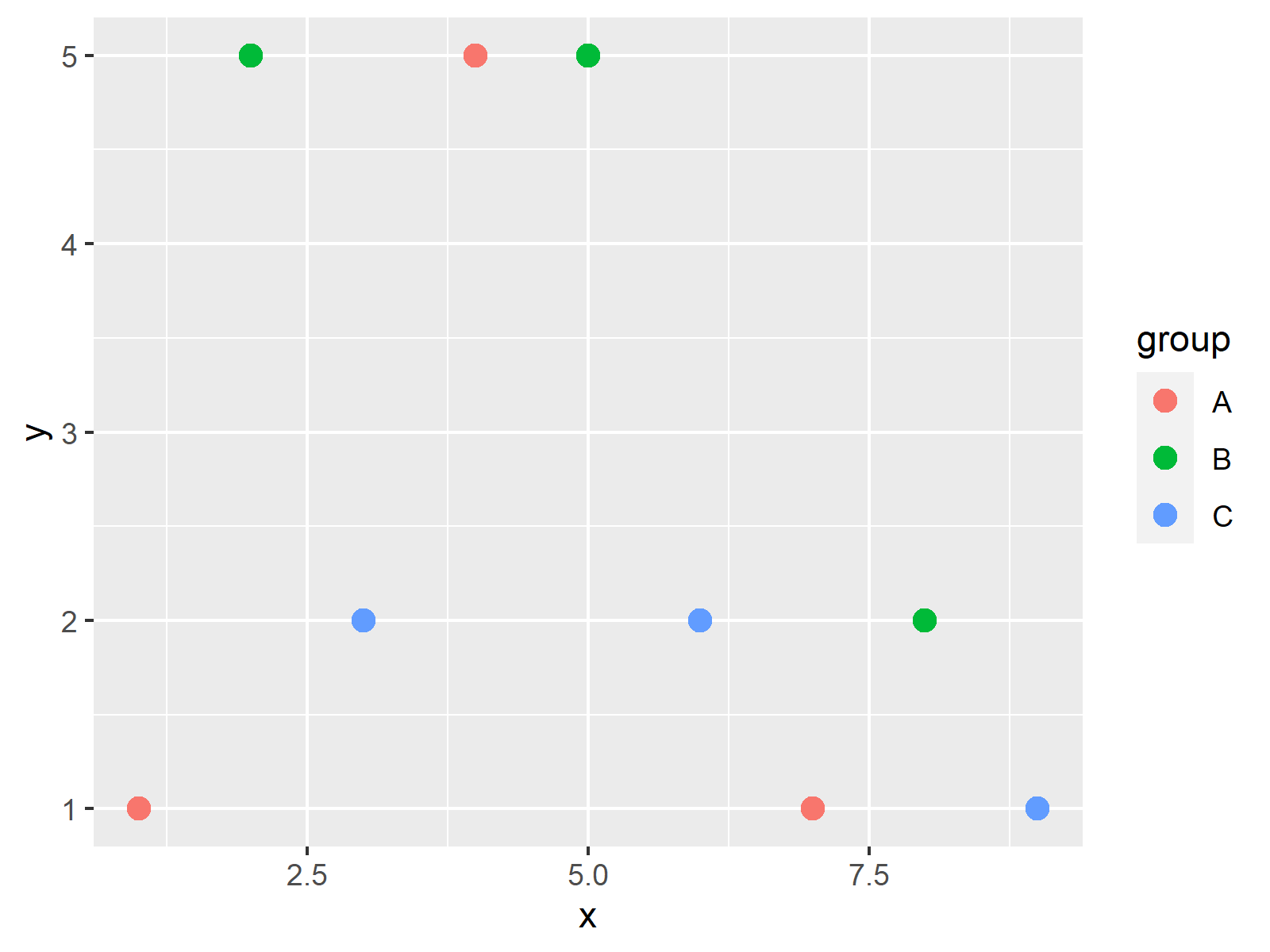As shown in Figure 1, we have created a new ggplot2 scatterplot.

Next, let’s create a contingency table based on the columns y and group:

```my_table <- as.data.frame(table(data[ , c(2, 3)]))  # Create example table
my_table                                            # Print example table```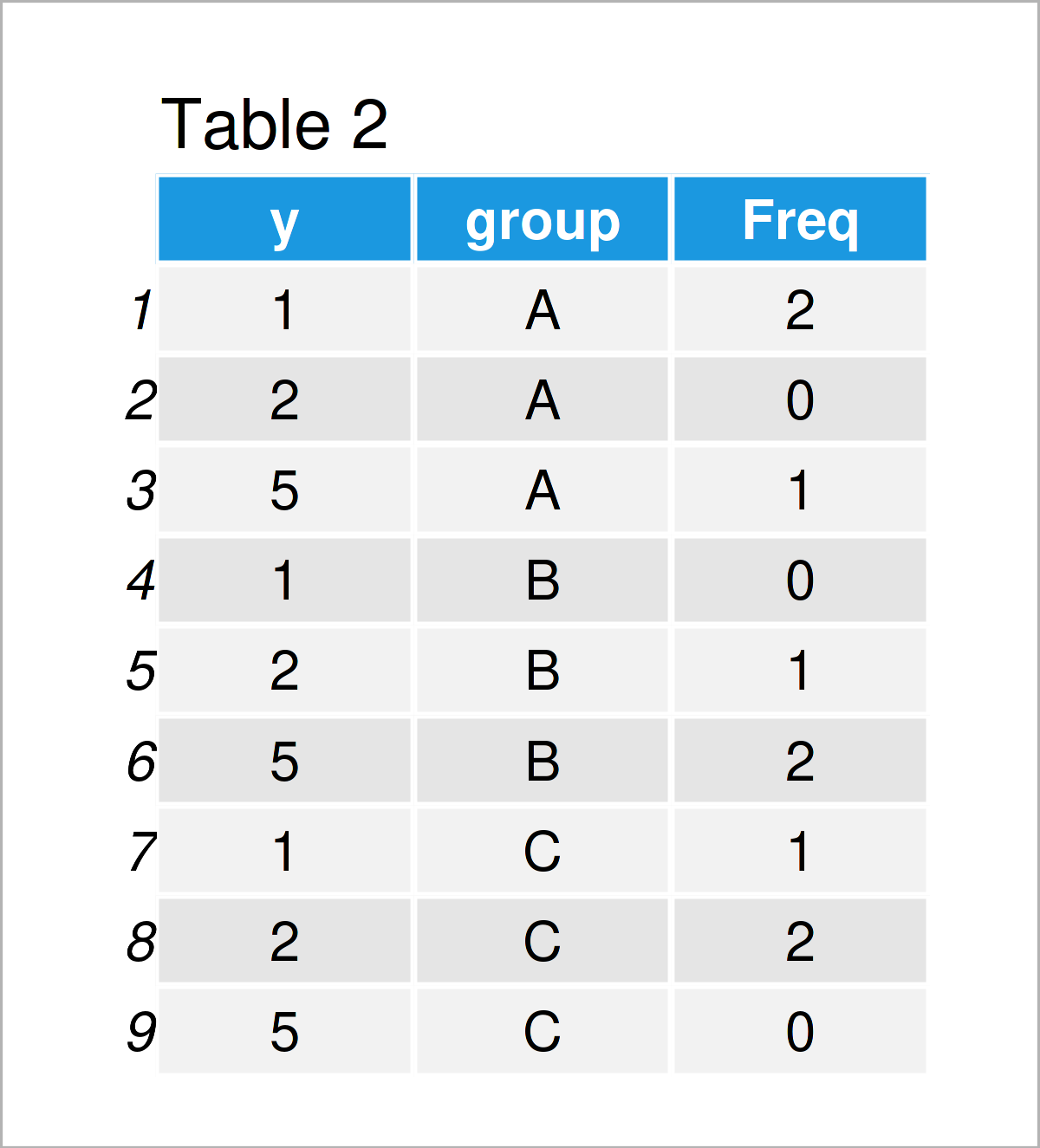As shown in Table 2, we have created a new contingency table that shows the frequencies of the combinations of our variables y and group.

In the next step, we have to create a plot object that contains only this table.

For this, we need to install and load the ggpmisc package:

```install.packages("ggpmisc")                         # Install & load ggpmisc package
library("ggpmisc")```

Next, we can draw our table within an empty ggplot2 plot:

```ggp_table <- ggplot() +                             # Create empty plot with table
theme_void() +
annotate(geom = "table",
x = 1,
y = 1,
label = list(my_table))
ggp_table                                           # Draw empty plot with table```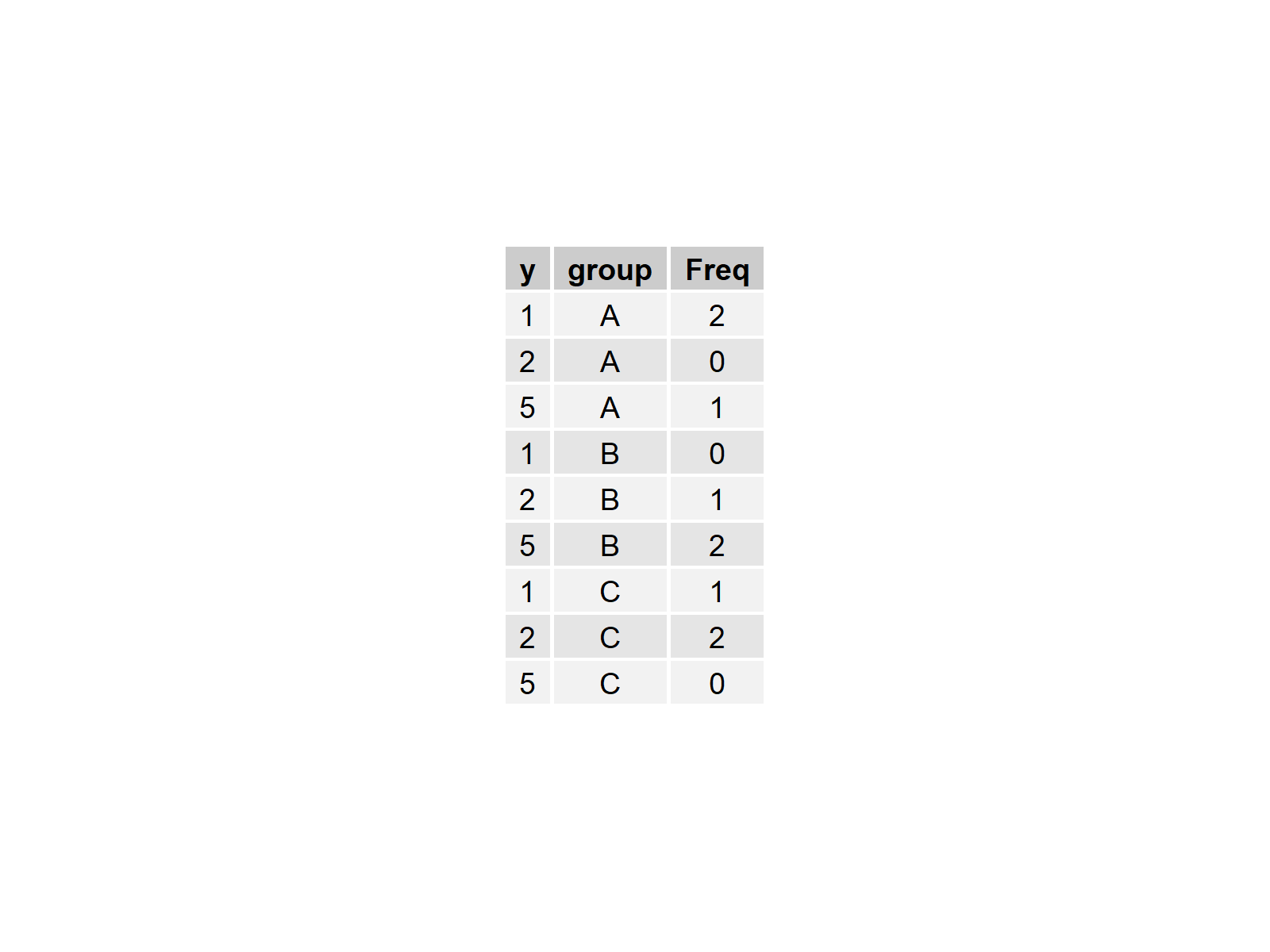The previous figure shows our empty plot with our contingency table inside.

Now, we can combine our plot and table in a grid layout using the patchwork package.

First, we need to install and load the patchwork package:

```install.packages("patchwork")                       # Install & load patchwork
library("patchwork")```

Next, we may draw our plot and table horizontally side-by-side to each other:

`ggp + ggp_table                                     # Plot and table side-by-side`We may also combine our plot and table vertically:

`ggp / ggp_table                                     # Combine plot and table vertically`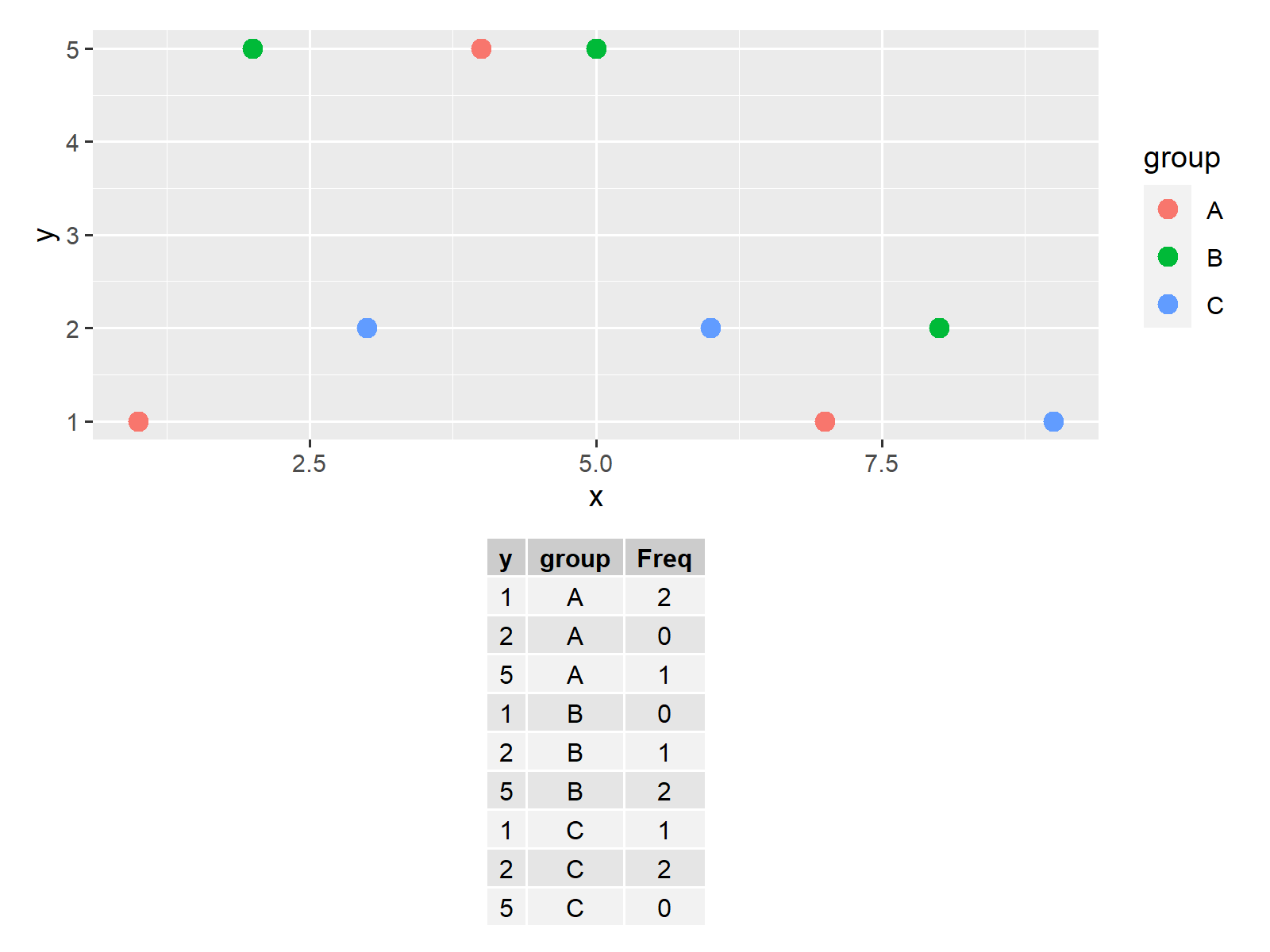Note that we could add even more tables and graphics to this layout.

## Video, Further Resources & Summary

In case you need more explanations on the R programming syntax of this article, I recommend having a look at the following video on my YouTube channel. In the video tutorial, I show the R syntax of this article in RStudio:

Furthermore, you could read some of the related tutorials on my website. Some tutorials about similar topics such as time objects, graphics in R, and plot legends are listed below.

To summarize: In this tutorial you have learned how to combine plots and tables in the same grid layout in the R programming language. If you have further questions, let me know in the comments. Furthermore, don’t forget to subscribe to my email newsletter to receive updates on the newest tutorials.

Subscribe to the Statistics Globe Newsletter